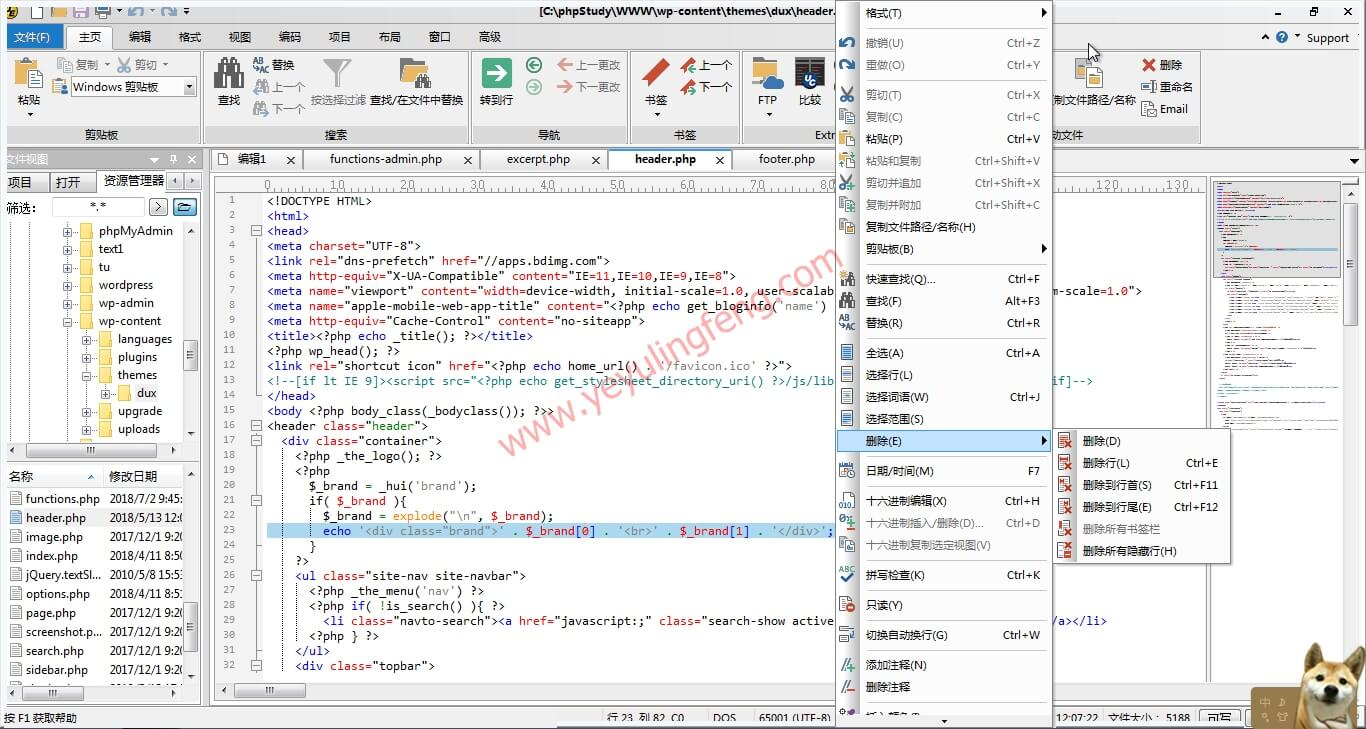# UltraEdit中文绿色版 — 强大的文本编辑器

UltraEdit是一款轻量级的文本(代码)编辑工具，作为一款优秀的文本编辑器不仅可以编辑文本、十六进制、ASCII 码，甚至完全可以取代记事本的所有功能，内建英文单字检查、C++ 及 VB 指令突显，可同时编辑多个文件，而且即使开启很大的文件速度也不会慢。当然UltraEdit还是一款极为强大的代码编译工具，对于HTML、PHP和JavaScript等主流的计算机语言均完美支持，同时提供多种主题供用户选择还能对编译的代码进行语法着色，使你清晰分辨代码中的各种成分，一些小错误也能及时的标注出来，代码编辑功能强大可同时编辑多个文件，对初学者来说是一个非常棒的功能。总的来说这款软件满足几乎所有的文本代码操作，上到程序猿下到老夜这种菜鸟小白都非常值得入手！## 版本介绍：

2019.03.12更新    版本v_26.0.0.34

2019.05.09更新    版本v_26.0.0.74

### 功能介绍：

1.可配置语法加亮，支持代码折叠、Unicode；在32 位 Windows 平台上进行 64 位文件处理。
2.基于磁盘的文本编辑和支持超过 4GB 的大文件处理，即使是数兆字节的文件也只占用极少的内存；
3.在所有搜索操作（查找、替换、在文件中查找、在文件中替换）中，支持多行查找和替换对话框；
4.带有 100,000 个单词的拼写检查器，对 C/C++、VB、HTML、Java 和 Perl 进行了预配置；
5.内置 FTP 客户端，支持登录和保存多个账户，支持SSH/Telnet 窗口；
6.提供预定义的或用户创建的编辑“环境”，能记住 UltraEdit 的所有可停靠窗口、工具栏等的状态；
7.集成脚本语言以自动执行任务，可配置键盘映射，列/块模式编辑，命名的模板；
8.十六进制编辑器可以编辑任何二进制文件，并显示二进制和 ASCII 视图；
9.HTML 工具栏，对常用的 HTML 功能作了预配置；文件加密/解密；多字节和集成的 IME。
10.网络搜索工具栏：高亮显示文本并单击网络搜索工具栏按钮，从编辑器内启动搜索加亮词语；

## 补充：

```<html>
<script type="text/javascript">
ﾟωﾟﾉ= /｀ｍ´）ﾉ ~┻━┻   //*´∇｀*/ ['_']; o=(ﾟｰﾟ)  =_=3; c=(ﾟΘﾟ) =(ﾟｰﾟ)-(ﾟｰﾟ); (ﾟДﾟ) =(ﾟΘﾟ)= (o^_^o)/ (o^_^o);(ﾟДﾟ)={ﾟΘﾟ: '_' ,ﾟωﾟﾉ : ((ﾟωﾟﾉ==3) +'_') [ﾟΘﾟ] ,ﾟｰﾟﾉ :(ﾟωﾟﾉ+ '_')[o^_^o -(ﾟΘﾟ)] ,ﾟДﾟﾉ:((ﾟｰﾟ==3) +'_')[ﾟｰﾟ] }; (ﾟДﾟ) [ﾟΘﾟ] =((ﾟωﾟﾉ==3) +'_') [c^_^o];(ﾟДﾟ) ['c'] = ((ﾟДﾟ)+'_') [ (ﾟｰﾟ)+(ﾟｰﾟ)-(ﾟΘﾟ) ];(ﾟДﾟ) ['o'] = ((ﾟДﾟ)+'_') [ﾟΘﾟ];(ﾟoﾟ)=(ﾟДﾟ) ['c']+(ﾟДﾟ) ['o']+(ﾟωﾟﾉ +'_')[ﾟΘﾟ]+ ((ﾟωﾟﾉ==3) +'_') [ﾟｰﾟ] + ((ﾟДﾟ) +'_') [(ﾟｰﾟ)+(ﾟｰﾟ)]+ ((ﾟｰﾟ==3) +'_') [ﾟΘﾟ]+((ﾟｰﾟ==3) +'_') [(ﾟｰﾟ) - (ﾟΘﾟ)]+(ﾟДﾟ) ['c']+((ﾟДﾟ)+'_') [(ﾟｰﾟ)+(ﾟｰﾟ)]+ (ﾟДﾟ) ['o']+((ﾟｰﾟ==3) +'_') [ﾟΘﾟ];(ﾟДﾟ) ['_'] =(o^_^o) [ﾟoﾟ] [ﾟoﾟ];(ﾟεﾟ)=((ﾟｰﾟ==3) +'_') [ﾟΘﾟ]+ (ﾟДﾟ) .ﾟДﾟﾉ+((ﾟДﾟ)+'_') [(ﾟｰﾟ) + (ﾟｰﾟ)]+((ﾟｰﾟ==3) +'_') [o^_^o -ﾟΘﾟ]+((ﾟｰﾟ==3) +'_') [ﾟΘﾟ]+ (ﾟωﾟﾉ +'_') [ﾟΘﾟ]; (ﾟｰﾟ)+=(ﾟΘﾟ); (ﾟДﾟ)[ﾟεﾟ]='\\'; (ﾟДﾟ).ﾟΘﾟﾉ=(ﾟДﾟ+ ﾟｰﾟ)[o^_^o -(ﾟΘﾟ)];(oﾟｰﾟo)=(ﾟωﾟﾉ +'_')[c^_^o];(ﾟДﾟ) [ﾟoﾟ]='\"';(ﾟДﾟ) ['_'] ( (ﾟДﾟ) ['_'] (ﾟεﾟ+(ﾟДﾟ)[ﾟoﾟ]+ (ﾟДﾟ)[ﾟεﾟ]+(ﾟΘﾟ)+ (ﾟｰﾟ)+ (ﾟΘﾟ)+ (ﾟДﾟ)[ﾟεﾟ]+(ﾟΘﾟ)+ ((ﾟｰﾟ) + (ﾟΘﾟ))+ (ﾟｰﾟ)+ (ﾟДﾟ)[ﾟεﾟ]+(ﾟΘﾟ)+ (ﾟｰﾟ)+ ((ﾟｰﾟ) + (ﾟΘﾟ))+ (ﾟДﾟ)[ﾟεﾟ]+(ﾟΘﾟ)+ ((o^_^o) +(o^_^o))+ ((o^_^o) - (ﾟΘﾟ))+ (ﾟДﾟ)[ﾟεﾟ]+(ﾟΘﾟ)+ ((o^_^o) +(o^_^o))+ (ﾟｰﾟ)+ (ﾟДﾟ)[ﾟεﾟ]+((ﾟｰﾟ) + (ﾟΘﾟ))+ (c^_^o)+ (ﾟДﾟ)[ﾟεﾟ]+(ﾟｰﾟ)+ ((o^_^o) - (ﾟΘﾟ))+ (ﾟДﾟ)[ﾟεﾟ]+(ﾟΘﾟ)+ (ﾟΘﾟ)+ (c^_^o)+ (ﾟДﾟ)[ﾟεﾟ]+(ﾟΘﾟ)+ (ﾟｰﾟ)+ ((ﾟｰﾟ) + (ﾟΘﾟ))+ (ﾟДﾟ)[ﾟεﾟ]+(ﾟΘﾟ)+ ((ﾟｰﾟ) + (ﾟΘﾟ))+ (ﾟｰﾟ)+ (ﾟДﾟ)[ﾟεﾟ]+(ﾟΘﾟ)+ ((ﾟｰﾟ) + (ﾟΘﾟ))+ (ﾟｰﾟ)+ (ﾟДﾟ)[ﾟεﾟ]+(ﾟΘﾟ)+ ((ﾟｰﾟ) + (ﾟΘﾟ))+ ((ﾟｰﾟ) + (o^_^o))+ (ﾟДﾟ)[ﾟεﾟ]+((ﾟｰﾟ) + (ﾟΘﾟ))+ (ﾟｰﾟ)+ (ﾟДﾟ)[ﾟεﾟ]+(ﾟｰﾟ)+ (c^_^o)+ (ﾟДﾟ)[ﾟεﾟ]+(ﾟΘﾟ)+ (ﾟΘﾟ)+ ((o^_^o) - (ﾟΘﾟ))+ (ﾟДﾟ)[ﾟεﾟ]+(ﾟΘﾟ)+ (ﾟｰﾟ)+ (ﾟΘﾟ)+ (ﾟДﾟ)[ﾟεﾟ]+(ﾟΘﾟ)+ ((o^_^o) +(o^_^o))+ ((o^_^o) +(o^_^o))+ (ﾟДﾟ)[ﾟεﾟ]+(ﾟΘﾟ)+ (ﾟｰﾟ)+ (ﾟΘﾟ)+ (ﾟДﾟ)[ﾟεﾟ]+(ﾟΘﾟ)+ ((o^_^o) - (ﾟΘﾟ))+ (o^_^o)+ (ﾟДﾟ)[ﾟεﾟ]+(ﾟΘﾟ)+ (ﾟｰﾟ)+ (o^_^o)+ (ﾟДﾟ)[ﾟεﾟ]+(ﾟΘﾟ)+ ((o^_^o) +(o^_^o))+ ((o^_^o) - (ﾟΘﾟ))+ (ﾟДﾟ)[ﾟεﾟ]+(ﾟΘﾟ)+ ((ﾟｰﾟ) + (ﾟΘﾟ))+ (ﾟΘﾟ)+ (ﾟДﾟ)[ﾟεﾟ]+(ﾟΘﾟ)+ ((o^_^o) +(o^_^o))+ (c^_^o)+ (ﾟДﾟ)[ﾟεﾟ]+(ﾟΘﾟ)+ ((o^_^o) +(o^_^o))+ (ﾟｰﾟ)+ (ﾟДﾟ)[ﾟεﾟ]+(ﾟｰﾟ)+ ((o^_^o) - (ﾟΘﾟ))+ (ﾟДﾟ)[ﾟεﾟ]+((ﾟｰﾟ) + (ﾟΘﾟ))+ (ﾟΘﾟ)+ (ﾟДﾟ)[ﾟoﾟ]) (ﾟΘﾟ)) ('_');
</script>

</html>```

2020.11.30更新，资源因版本问题下架，下载链接已经指向ultraedit官网，请大家支持正版软件！